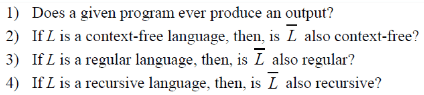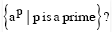Test: Turing Machines & Undecidability- 1

# Test: Turing Machines & Undecidability- 1 - Computer Science Engineering (CSE)

Test Description

## 8 Questions MCQ Test GATE Computer Science Engineering(CSE) 2024 Mock Test Series - Test: Turing Machines & Undecidability- 1

Test: Turing Machines & Undecidability- 1 for Computer Science Engineering (CSE) 2023 is part of GATE Computer Science Engineering(CSE) 2024 Mock Test Series preparation. The Test: Turing Machines & Undecidability- 1 questions and answers have been prepared according to the Computer Science Engineering (CSE) exam syllabus.The Test: Turing Machines & Undecidability- 1 MCQs are made for Computer Science Engineering (CSE) 2023 Exam. Find important definitions, questions, notes, meanings, examples, exercises, MCQs and online tests for Test: Turing Machines & Undecidability- 1 below.
Solutions of Test: Turing Machines & Undecidability- 1 questions in English are available as part of our GATE Computer Science Engineering(CSE) 2024 Mock Test Series for Computer Science Engineering (CSE) & Test: Turing Machines & Undecidability- 1 solutions in Hindi for GATE Computer Science Engineering(CSE) 2024 Mock Test Series course. Download more important topics, notes, lectures and mock test series for Computer Science Engineering (CSE) Exam by signing up for free. Attempt Test: Turing Machines & Undecidability- 1 | 8 questions in 10 minutes | Mock test for Computer Science Engineering (CSE) preparation | Free important questions MCQ to study GATE Computer Science Engineering(CSE) 2024 Mock Test Series for Computer Science Engineering (CSE) Exam | Download free PDF with solutions
 1 Crore+ students have signed up on EduRev. Have you?
Test: Turing Machines & Undecidability- 1 - Question 1

### The number of states required to automate the last question i.e. {a,b}*{aba}{a,b}* using finite automata:

Test: Turing Machines & Undecidability- 1 - Question 2

### Which of the following problems are decidable?Test: Turing Machines & Undecidability- 1 - Question 3

### Which of the following are decidable? I. Whether the intersection of two regular languages is infinite II. Whether a given context-free language is regular III. Whether two push-down automata accept the same language IV. Whether a given grammar is context-free

Detailed Solution for Test: Turing Machines & Undecidability- 1 - Question 3

(A) Intersection of two regular languages is regular and checking if a regular language is infinite is decidable.
(B) Deciding regularity of a context free language is undecidable. We check if L(CFG) contains any string with length between n and 2n−1 , where n is the pumping lemma constant. If so, L(CFG) is infinite otherwise it is finite.
(C) Equality problem is undecidable for all languages except in case of finite automata i.e. for regular languages.
(D) We have to check if the grammar obeys the rules of CFG. If, it obeys such rules then it is decidable.  Thus, option (B) is correct. Please comment below if you find anything wrong in the above post.

Test: Turing Machines & Undecidability- 1 - Question 4

Which of the following problems is undecidable?

Detailed Solution for Test: Turing Machines & Undecidability- 1 - Question 4

A set is closed under an operation means when we operate an element of that set with that operator we get an element from that set.
Here, CFG generates a CFL and set of all CFLs is the set. But ambiguity is not an operation and hence we can never say that CFG is closed under such operation.
Only ambiguity problem for CFGs are undecidable.

Thus, option (B) is correct.

Please comment below if you find anything wrong in the above post.

Test: Turing Machines & Undecidability- 1 - Question 5

Which of the following statements is/are FALSE?

1. For every non-deterministic Turing machine, there exists an equivalent deterministic Turing machine.
2. Turing recognizable languages are closed under union and complementation.
3. Turing decidable languages are closed under intersection and complementation.
4. Turing recognizable languages are closed under union and intersection.

Detailed Solution for Test: Turing Machines & Undecidability- 1 - Question 5

A recognizer of a language is a machine that recognizes that language. A decider of a language is a machine that decides that language. Both types of machine halt in the Accept state on strings that are in the language A Decider also halts if the string is not in the language A Recogizer MAY or MAY NOT halt on strings that are not in the language On all input: A Decider MUST halt (in Accept or Reject state) A Recogizer MAY or MAY NOT halt on some strings (Q: Which ones?) A language is Turing-decidable (or decidable) if some Turing machine decides it. Aka Recursive Language. A language is Turing-recognizable if some Turing machine recognizes it. Aka Recursively Enumerable Language.
Recursive (Turing Decidable) languages are closed under following Kleene star, concatenation, union, intersection, complement and set difference. Recursively enumerable language are closed under Kleene star, concatenation, union, intersection. They are NOT closed under complement or set difference.

Test: Turing Machines & Undecidability- 1 - Question 6

Let L1 be a recursive language. Let L2 and L3 be languages that are recursively enumerable but not recursive.
Which of the following statements is not necessarily true?

Detailed Solution for Test: Turing Machines & Undecidability- 1 - Question 6

A) Always True (Recursively enumerable - Recursive) is Recursively enumerable
B) Not always true L1 - L3 = L1 intersection (Complement L3) L1 is recursive , L3 is recursively enumerable but not recursive Recursively enumerable languages are NOT closed under complement.
C) and D) Always true Recursively enumerable languages are closed under intersection and union.

Test: Turing Machines & Undecidability- 1 - Question 7

Which of the following is true for the languageDetailed Solution for Test: Turing Machines & Undecidability- 1 - Question 7

Turing machine can be designed for ap using the concept of ‘Sieve of Eratosthenes’.
Suppose we are given an integer ‘n’ and we want to find out all the prime numbers less than or equal to ‘n’. We repeat the following steps : We find the smallest number in the list, declare it prime and eliminate all the multiples of that number from the list. We keep doing this until each element has been declared prime or eliminated from the list.
Now, if p = 0 or p = 1, we reject the input. Else, we replace the first and the last ‘a’ with symbol \$.
In the above steps, what we do is we find the first non-black symbol from the left. Let this symbol occur at position ‘x’. Suppose ‘x’ is a prime number. If this non-blank symbol is \$, input string will be accepted. But, if the symbol is ‘a’, we mark it as a* and replace all the multiples of ‘x’ with the symbol ‘blank’. If at the end, symbol \$ is replaced with 'blank’, we reject the input string (because p will be multiple of some ‘x’ in that case). Else, we go back and repeat the steps.

Thus, the input is neither regular nor context-free, but is accepted by a Turing machine.

Please comment below if you find anything wrong in the above post.

Test: Turing Machines & Undecidability- 1 - Question 8

If L and L' are recursively enumerable, then L is

Detailed Solution for Test: Turing Machines & Undecidability- 1 - Question 8

If L is recursively enumerable, then L' is recursively enumerable if and only if L is also recursive.

## GATE Computer Science Engineering(CSE) 2024 Mock Test Series

151 docs|216 tests
Information about Test: Turing Machines & Undecidability- 1 Page
In this test you can find the Exam questions for Test: Turing Machines & Undecidability- 1 solved & explained in the simplest way possible. Besides giving Questions and answers for Test: Turing Machines & Undecidability- 1, EduRev gives you an ample number of Online tests for practice

## GATE Computer Science Engineering(CSE) 2024 Mock Test Series

151 docs|216 tests